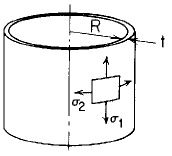Related Resources: pressure-vessel

### Cylinder Stress and Deflection by Own Weight by Own Weight Equation and Calculator

Cylinder by Own Weight, δ = force top edge support, bottom edge free Stress and Deflection Equation and Calculator.

Per. Roarks Formulas for Stress and Strain for membrane stresses and deformations in thin-walled pressure vessels.

 Cylindrical( R / t ) > 10

When:
R / t > 10

Meridional Stress at top
σ1 = δy

Circumferential or Hoop Stress
σ2 = 0Change in height dimension yRotation of a meridian from its unloaded positionWhere used:
E = Modulus of Elasticity (lbs/in2)
v = Poisson's ratio
σ1,2 = stress, (lbs/in2)
t = Cylinder thickness, (in)
y = Length of cylinder, (in)
δ = Mass per section (lbs/in2)
ψ = Rotation of a meridian from its unloaded position, positive when that meridional rotation represents an increase in ΔR when y or θ increases;

Reference:

Roarks Formulas for Stress and Strain, 7th Edition